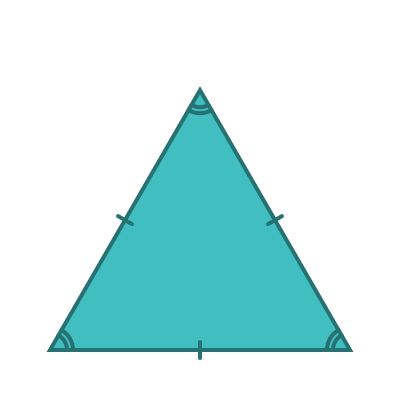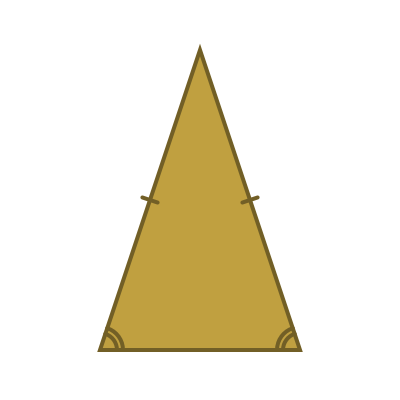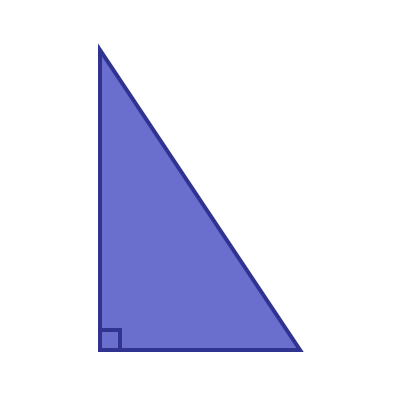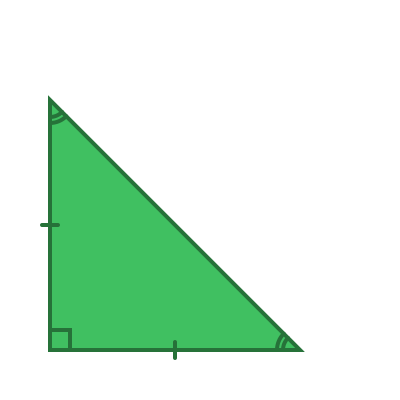# Triangles

Martin McBride, 2020-08-14
Tags triangle equilateral triangle isosceles triangle scalene triangle right angled triangle
Categories maths gcse geometry

A triangle is a shape with three straight sides. There are several types of triangle. Here is a video that describes them:

## Equilateral triangles

An equilateral triangle is a triangle where all three sides are the same length:The tick marks on the three sides indicate that the sides are the same length.

The three angles of an equilateral triangle are also all equal. The angle arcs in the corners indicate this.

The three angles of any triangle always add up to 180 degrees. It follows that each angle in an equilateral triangle must be equal to 60 degrees.

## Isosceles triangles

An isosceles triangle has two sides that are equal in length. The third side is not equal to the other two (it can be longer or shorter).We sometimes call the two equal sides the legs of the triangle, and the other side the base.

Each leg makes an equal angle with the base.

## Scalene triangles

A scalene triangle has three unequal sides.Al three angles in a scalene triangle are unequal.

## Obtuse and acute triangles

A triangle is acute if all three of its angles are less than 90 degrees. The isosceles triangle above is acute.

A triangle is obtuse is one of its angles is greater than 90 degrees. The scalene triangle above is obtuse.

It is impossible for a triangle to have more than one angle that is greater than 90 degrees, because the sum of all three angles is 180 degrees.

## Right-angled triangle

A right-angled triangle has one angle of exactly 90 degrees.The sum of the other two angles is 90 degrees.

Every triangle is either acute, right-angled or obtuse.

## Right-angled isosceles triangle

A right-angled isosceles triangle has one angle of 90 degrees, and two equal sides.Since we know that the two remaining angles are equal for an isosceles triangle, we know that they must be exactly 45 degrees, so that the sum of all three angles is 90 + 45 + 45 = 180 degrees.